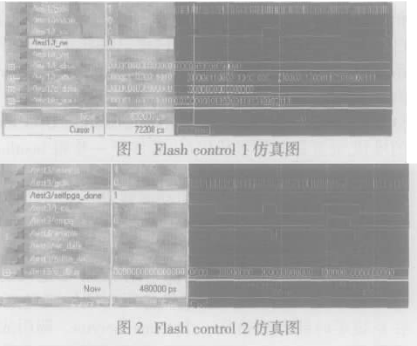## 采用VHDL语言在CPLD内部编程实现Flash读取控制设计2020-07-25 23:08:11    评分

1、概述

2、实现

2．1 器件的选择

2．2 实现中的问题及解决方法

2．3 基本设计模块图

2．4 用VHDL进行实现（注：实体部分定义可分别参见模块图中的划分）

Flash control 1

process（reset，gclk）

begin

if reset =”0“ then

count_reset 《=”0“;

elsif gclk”event and gclk =“1” then

count_reset 《= not（count（4） and count（3） and count（2））;

end if;

end process;

process（count_reset，gclk）

begin

if count_reset =“0” then

count 《=（others =》“0”）;

elsif gclk“event and gclk =”1“ then

count 《=count +1;

end if;

end process;

process（count_reset，reset，enable）

begin

if reset =”0“ then

F_CS 《=”1“;

F_OE 《=”1“;

F_WE 《=”1“;

elsif count_reset =”0“ then

F_CS 《= ”1“;

F_OE 《= ”1“;

F_WE 《= ”1“;

elsif enable”event and enable =“1” then

F_Abus 《= C_Abus;

F_CS 《= “0”;

F_OE 《= “0”;

F_WE 《= “1”;

C_Dbus 《= F_Dbus;

end if;

end process;

Flash control 2

d_count_rst 《= not（d_count（1） and （not d_count（0）） and empty）;

sig_WD 《= not（d_count（1） and （not d_count（0）））;

WR_DATA 《= sig_WD;

process（gclk，d_count）

begin

if reset =“0” then

d_count 《= “1”;

elsif F_CS = “1” then

d_count1 《= not d_count1 ;

if gclk“event and gclk =”1“ then

d_count2 《= d_count1;

d_count 《= d_count2;

end if;

end if;

end process;

process（reset，d_count，C_Dbus）

begin

if reset = ”0“ then

data_adder 《= （others =》”0“）;

elsif d_count”event and d_count = “0” then

data_adder（31 downto 16） 《= C_Dbus;

elsif d_count“event and d_count = ”1“ then

data_adder（15 downto 0） 《= C_Dbus;

end if;

end process;

enable 《= enable1 and enable2;

process（reset，d_count，F_CS）

begin

if reset = ”0“ or F_CS = ”0“ then

enable1 《= ”1“;

enable2 《= ”1“;

elsif d_count”event then

if gclk“event and gclk =”1“ then

enable1 《= ”0“;

enable2 《= not enable1;

end if;

end if;

end process;

process（reset，F_CS）

begin

if reset = ”0“ then

address《= X”400000“;

elsif F_CS = ”1“ then

C_Abus 《= address;

address 《= adderss +1;

end if;

end process;

process（reset，F_CS）

begin

if reset = ”0“ then

address《= X”400000“;

data_adder 《=（others =》”0“）;

elsif F_CS = ”1“;

address 《= adderss +1;

end if;

end process;

process（d_count2，F_CS）

begin

if F_CS = ”0“ then

enable1 《= ”1“;

enable 《= ”1“;

elsif d_count2 = ”00“ or d_count2 = ”01“ then

enable 《= ”0“;

end if;

end process;

process（gclk，sig_WD）

begin

if sig_WD = ”1“ then

Pulse_RW 《=”1“;

elsif gclk”event and gclk =“1” then

W_D1 《= sig_WD;

W_D2 《= W_D1;

if empty =“0” then

data_adder1 《= data_adder;

Pulse_RW 《= （W_D1 or （not W_D2））;

end if;

end if;

end process;

（注：此程序在Xilinx公司的ISE6.2i环境下用VHDL编程实现，其仿真波形见下图）3、 结束语

（注：此程序在Xilinx公司的ISE6.2i环境下用VHDL编程实现，其仿真波形见下图）

3 、结束语2020-07-26 18:09:00    评分
2楼2020-07-26 18:12:33    评分
3楼2020-07-26 18:15:47    评分
4楼2020-07-26 18:19:31    评分
5楼2020-07-26 18:22:28    评分
6楼2020-07-26 18:26:56    评分
7楼2020-07-26 22:59:59    评分
8楼